Grade - examples - page 169

1. Trapezoid - intersection of diagonalsIn the ABCD trapezoid is AB = 8 cm long, trapezium height 6 cm, and distance of diagonals intersection from AB is 4 cm. Calculate trapezoid area.
2. Unknown number 17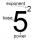Milada said, I am thinking of a number such that I evaluate expression x1/3, the value of the expression would be 5. Which number Milada thinking?
3. Eq1Solve equation: 4(a-3)=3(2a-5)
4. Box and whisker plotConstruct a box and whisker plot for the given data. 56, 32, 54, 32, 23, 67, 23, 45, 12, 32, 34, 24, 36, 47, 19, 43
5. If-then equationIf 5x - 17 = -x + 7, then x =
6. Circle - AGFind the coordinates of circle and its diameter if its equation is: ?
7. Family 8Father is 38 years old, daughter 12, son 14. How many years will father have as many years as his children together?
8. Lcd3What is LCD of the equation of ? ? And what is x?
9. Photo enlarge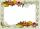Mark wants to enlarge a 4-inch by 6-inch photo so that it has a height of 15inches. Use a ratio table to determine the new width of the photo.
10. CandyPeter had a sachet of candy. He wanted to share with his friends. If he gave them 30 candies, he would have 62 candies. If he gave them 40 candies, he would miss 8 candies. How many friends did Peter have?
11. Equation of circlefind an equation of the circle with indicated properties: a. center at (-3,5), diameter 20. b. center at origin and diameter 16.
12. How far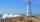From the top of a lighthouse 145 ft above sea level, the angle of depression of a boat 29°. How far is the boat from the lighthouse?
13. Fridays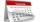Friday 13th is in 4 days. What day is today and what day it is?
14. Unknown numbersThe sum of two consecutive natural numbers and their triple is 92. Find these numbers.
15. Clubhouse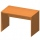There were only chairs and table in the clubhouse. Each chair had four legs, and the table was triple. Scouts came to the clubhouse. Everyone sat on their chair, two chairs were left unoccupied, and the number of legs in the room was 101. How many chairs w
16. Two cuboidsFind the volume of cuboidal box whose one edge is: a) 1.4m and b) 2.1dm
17. Rhombus constructionConstruct ABCD rhombus if its diagonal AC=9 cm and side AB = 6 cm. Inscribe a circle in it touching all sides...
18. Five pumpsThe water tank is filled with two pumps in 48 minutes. How long would it take to fill it with 5 same pumps?
19. CompetitorsIn the first round of slalom fell 15% of all competitors and in the second round another 10 racers. Together, 40% of all competitors fell. What was the total number of competitors?
20. MultiplesFind all multiples of 10 that are larger than 136 and smaller than 214.

Do you have an interesting mathematical example that you can't solve it? Enter it, and we can try to solve it.

To this e-mail address, we will reply solution; solved examples are also published here. Please enter e-mail correctly and check whether you don't have a full mailbox.